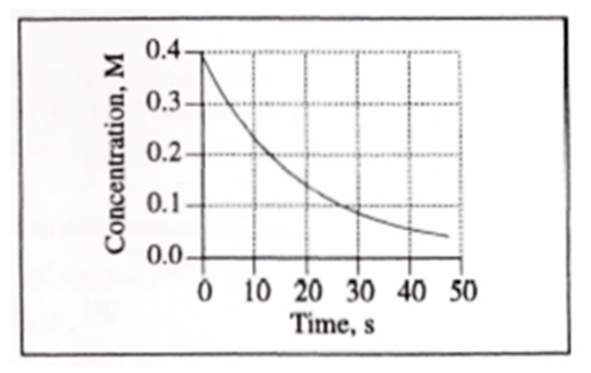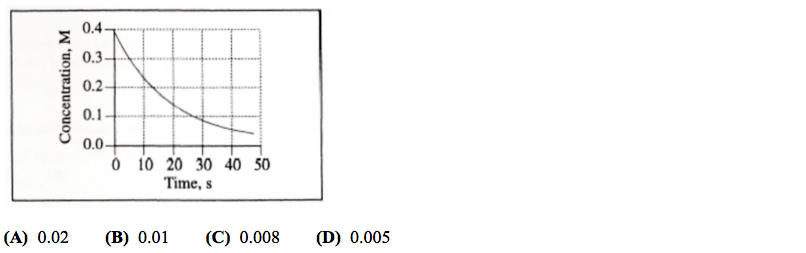# Problem: What is the initial rate of the reaction (in mol•L•s –1) depicted by this graph?

###### FREE Expert Solution
89% (431 ratings)
###### FREE Expert Solution

We’re being asked for the initial rate of the reaction (in molL-1s-1) based on the graph given.

An instantaneous reaction rate is the rate at a particular moment in the reaction and is usually determined graphically and can be calculated as:

Δ[  ] = change in concentration
Δt = change in time

For the initial rate, we’ll look at the smallest time possible and determine the concentration at that time. The graph below has a 10-second increments.89% (431 ratings)###### Problem Details

What is the initial rate of the reaction (in mol•L•s –1) depicted by this graph?Frequently Asked Questions

What scientific concept do you need to know in order to solve this problem?

Our tutors have indicated that to solve this problem you will need to apply the Instantaneous Rate of Change concept. If you need more Instantaneous Rate of Change practice, you can also practice Instantaneous Rate of Change practice problems.

What is the difficulty of this problem?

Our tutors rated the difficulty ofWhat is the initial rate of the reaction (in mol•L•s –1) dep...as high difficulty.

How long does this problem take to solve?

Our expert Chemistry tutor, Jules took 2 minutes and 34 seconds to solve this problem. You can follow their steps in the video explanation above.

What professor is this problem relevant for?

Based on our data, we think this problem is relevant for Professor Fleming's class at TEMPLE.